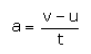# What is retardation? Write its formula. Given an example

What is retardation? Write its formula. Given an example where it acts on a body.

Retardation is a decrease in acceleration. This means that retardation is the rate of decrease in velocity. A positive sign of the magnitude of acceleration shows increase in velocity and a negative sign shows decrease in velocity.

Formula:Example: When brakes are applied to a moving bicycle, there is retardation in its motion.